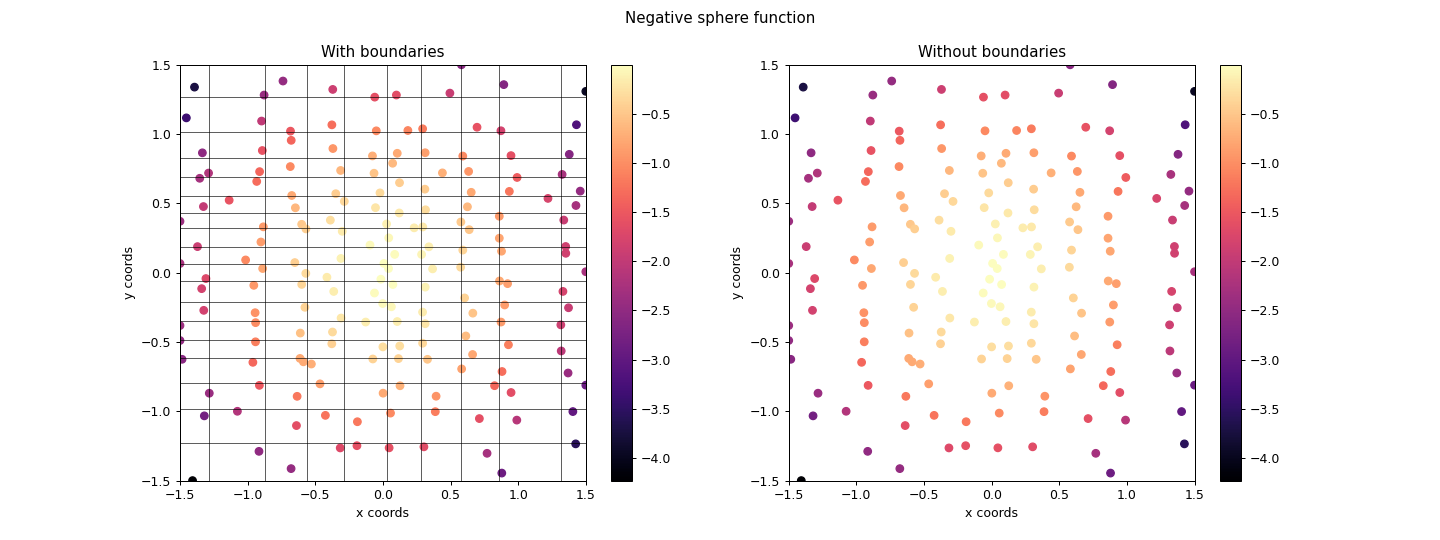# ribs.visualize.sliding_boundaries_archive_heatmap¶

ribs.visualize.sliding_boundaries_archive_heatmap(archive, ax=None, transpose_bcs=False, cmap='magma', square=False, ms=None, boundary_lw=0, vmin=None, vmax=None)[source]

Plots heatmap of a SlidingBoundariesArchive with 2D behavior space.

Since the boundaries of ribs.archives.SlidingBoundariesArchive are dynamic, we plot the heatmap as a scatter plot, in which each marker is an elite and its color represents the objective value. Boundaries can optionally be drawn by setting boundary_lw to a positive value.

Examples

>>> import numpy as np
>>> import matplotlib.pyplot as plt
>>> from ribs.archives import SlidingBoundariesArchive
>>> from ribs.visualize import sliding_boundaries_archive_heatmap
>>> archive = SlidingBoundariesArchive([10, 20],
...                                  [(-1, 1), (-1, 1)],
...                                  seed=42)
>>> archive.initialize(solution_dim=2)
>>> # Populate the archive with the negative sphere function.
>>> rng = np.random.default_rng(10)
>>> for _ in range(1000):
...     x, y = rng.uniform((-1, -1), (1, 1))
...         solution=np.array([x,y]),
...         objective_value=-(x**2 + y**2),
...         behavior_values=np.array([x, y]),
...     )
>>> # Plot heatmaps of the archive.
>>> fig, (ax1, ax2) = plt.subplots(1, 2, figsize=(16,6))
>>> fig.suptitle("Negative sphere function")
>>> sliding_boundaries_archive_heatmap(archive, ax=ax1,
...                                  boundary_lw=0.5)
>>> sliding_boundaries_archive_heatmap(archive, ax=ax2)
>>> ax1.set_title("With boundaries")
>>> ax2.set_title("Without boundaries")
>>> ax1.set(xlabel='x coords', ylabel='y coords')
>>> ax2.set(xlabel='x coords', ylabel='y coords')
>>> plt.show()Parameters
• archive (SlidingBoundariesArchive) – A 2D SlidingBoundariesArchive.

• ax (matplotlib.axes.Axes) – Axes on which to plot the heatmap. If None, the current axis will be used.

• transpose_bcs (bool) – By default, the first BC in the archive will appear along the x-axis, and the second will be along the y-axis. To switch this (i.e. to transpose the axes), set this to True.

• cmap (str, list, matplotlib.colors.Colormap) – Colormap to use when plotting intensity. Either the name of a colormap, a list of RGB or RGBA colors (i.e. an Nx3 or Nx4 array), or a colormap object.

• square (bool) – If True, set the axes aspect ratio to be “equal”.

• ms (float) – Marker size for the solutions.

• boundary_lw (float) – Line width when plotting the boundaries. Set to 0 to have no boundaries.

• vmin (float) – Minimum objective value to use in the plot. If None, the minimum objective value in the archive is used.

• vmax (float) – Maximum objective value to use in the plot. If None, the maximum objective value in the archive is used.

Raises

ValueError – The archive is not 2D.